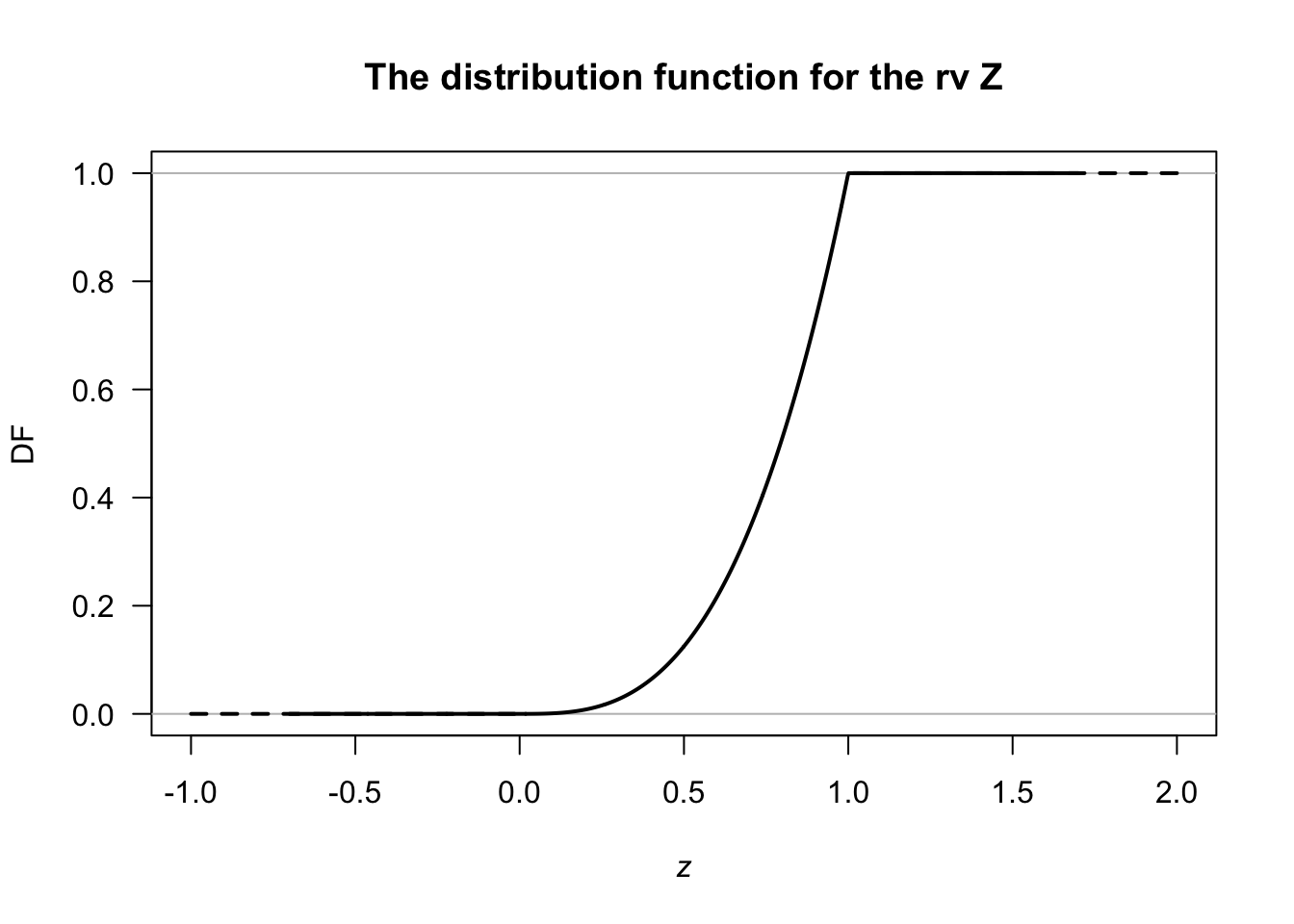## 3.1 CDF for continuous rvs

The CDF $$F_X(x)$$ for a continuous rv $$X$$ with PDF $$f_X(x)$$ is $F_X(x) = \Pr(T \le x) = \int_{-\infty}^x f(t)\,dt.$

Example 3.1 (CDF: continuous rv) From Example 2.3, $f_Z(z) = 3 z^2\quad\text{for 0<z<1}.$ The CDF is $F_Z(z) = \int_{-\infty}^z 3 t^2\, dt = \int_{0}^z 3 t^2\, dt = z^3.$ More completely and correctly, $F_Z(z) = \left\{ \begin{array}{ll} 0 & \text{for z<0}\\ z^3 & \text{for 0\le z \le 1}\\ 1 & \text{for z>1} \end{array} \right.;$ see Fig. 3.1.FIGURE 3.1: The distribution function for the variable $$Z$$